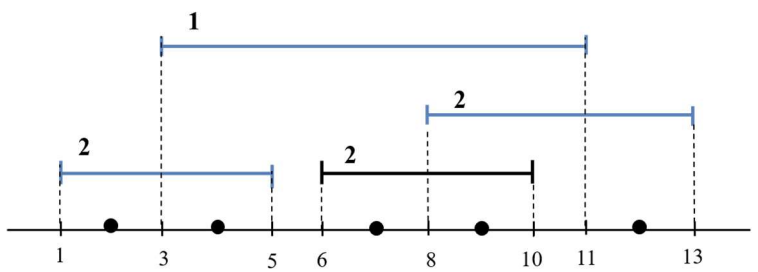시간 제한메모리 제한제출정답맞은 사람정답 비율
2 초 (추가 시간 없음) 1024 MB32121145.833%

## 문제

A farmer grows rare flowers, which are located on a line. The total number of flowers is $n$, and each flower $f_i$ has a location $x_i$ on the line. Each flower can be considered as a point on the line. In order to nourish the flowers, the farmer fertilizes them regularly. So the farmer will rent several fertilizer drones.

The drones spray fertilizer on the ground as they fly. The flight trajectory of each drone is a line segment, which can be considered as a closed interval. There are $m$ given drones $D_i$ with intervals $[a_i, b_i]$, each of which has a cost $c_i$. We should choose a set of drones such that each of the given points is contained in at least one of the chosen intervals, that is, the union of the chosen intervals contains all the points. The set of drones satisfying this condition is said to be a candidate set of drones.

We assume that all the points and the endpoints of all the intervals have distinct coordinates. We say that a point is covered by an interval, if it is contained in the interval. For a candidate set $X$ of drones, the cost paid to each point is defined to be the sum of the costs of intervals in $C$ which cover the point. Our goal is to find an optimal set of drones, minimizing the maximum cost of points among all the candidate sets.For example, there are five points on a line and four given intervals as shown in the above figure. In this figure, the numbers on the intervals represent their costs. All possible candidate sets of intervals are $\{[1, 5],[6, 10], [8, 13]\}, \{[1, 5],[3, 11], [8, 13]\}$, and the set with all of four intervals. For the first set, the point located at $9$ has the cost $4$, which is the maximum cost of points. For the second and the third set, the same point has the costs $3$ and $5$, respectively, which are the maximum. Thus the cost $3$ is the minimum of the maximum cost of points among all the candidate sets.

Given the locations of $n$ points and $m$ intervals with their costs, write a program to output the minimum of the maximum cost of points among all the candidate sets of drones.

## 입력

Your program is to read from standard input. The input starts with a line containing two integers, $n$ and $m$ ($n \ge 1$, $2 \le n + m \le 100,000$, $1 \le m \le 2,000$), where $n$ is the number of points and $m$ is the number of intervals. The second line contains $n$ integers, representing the locations of points, where the integers are between $0$ and $10^9$. In the following $m$ lines, each line contains three integers $a$, $b$, and $c$, representing an interval $[a, b]$ with a cost $c$ ($0 \le a < b \le 10^9$ and $1 \le c \le 10^9$). Note that all the points and the endpoints of all the intervals have distinct coordinates.

## 출력

Your program is to write to standard output. Print exactly one line. The line should contain the minimum of the maximum cost of points among all the candidate sets of drones. If there is no candidate set of drones, then the line should contain -1.

## 예제 입력 1

5 4
4 9 2 7 12
1 5 2
6 10 2
3 11 1
8 13 2


## 예제 출력 1

3


## 예제 입력 2

5 4
4 9 2 7 12
1 5 2
8 11 2
3 6 1
10 13 2


## 예제 출력 2

-1


## 예제 입력 3

18 7
3 4 6 13 14 8 9 11 22 23 16 17 18 29 30 31 26 27
5 21 2
19 24 6
7 15 3
2 12 4
20 28 5
1 10 5
25 32 3


## 예제 출력 3

6


## 출처

• 데이터를 추가한 사람: rdd6584